# Basic mechanical engineering(BMET-101/102) unit 2 (part-2) thermodynamics laws by varun pratap singh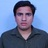Assistant Professor in ME Cluster @UPES um University of Petroleum and Energy Studies
15. Jul 2021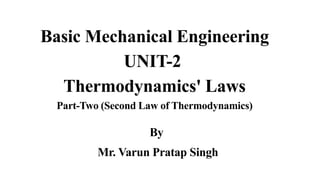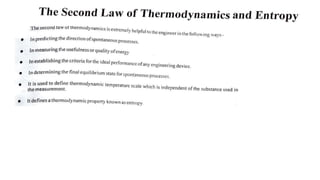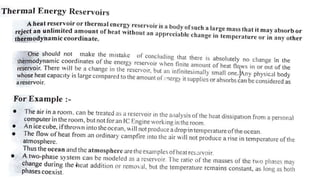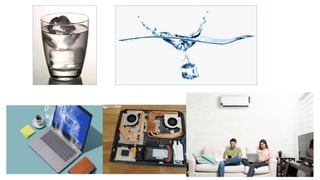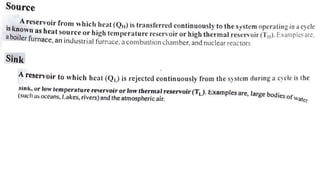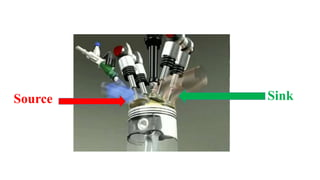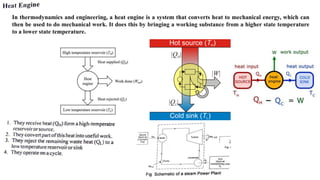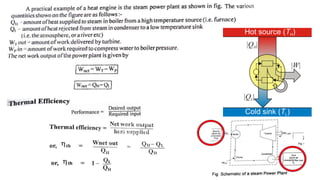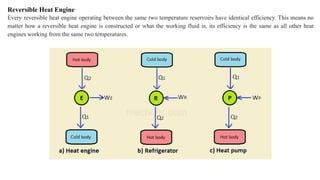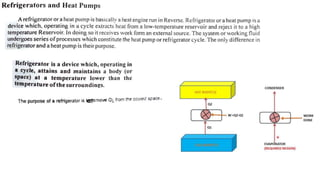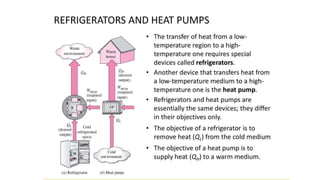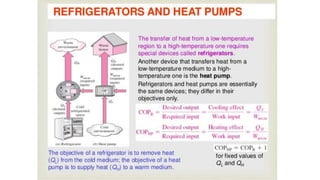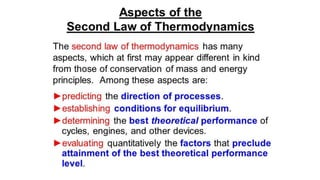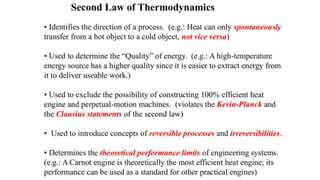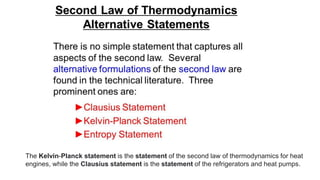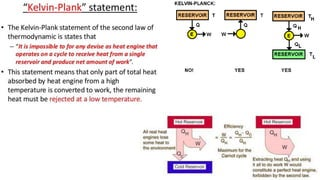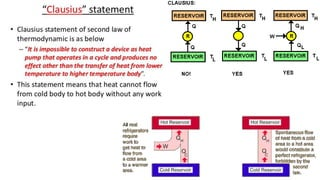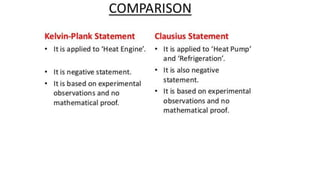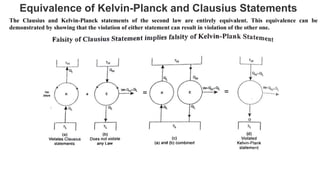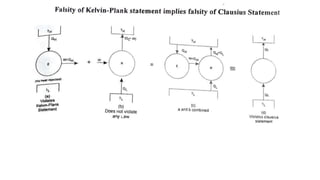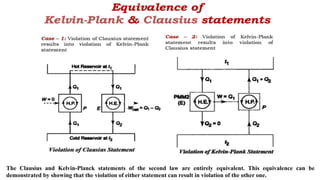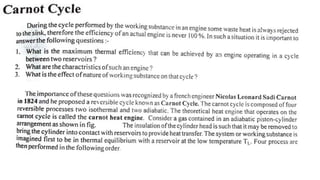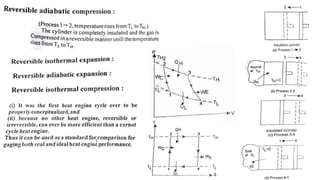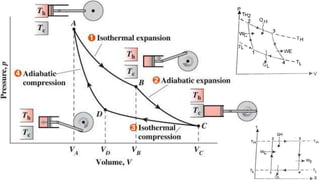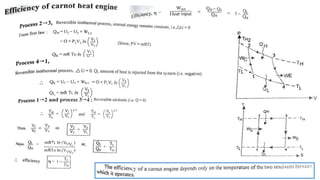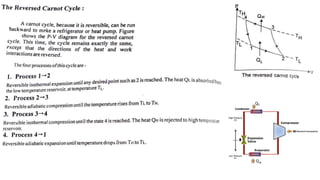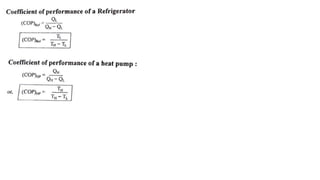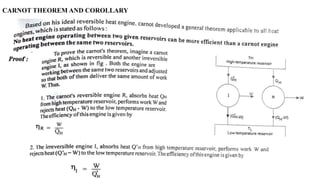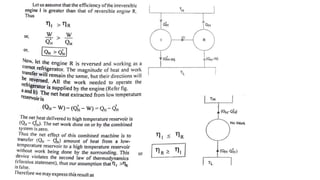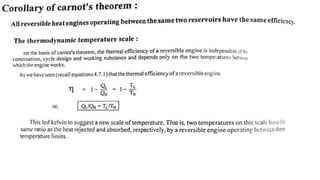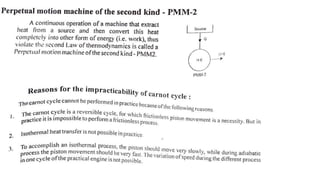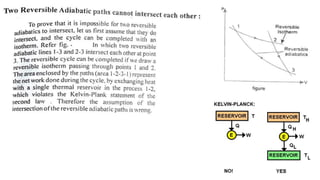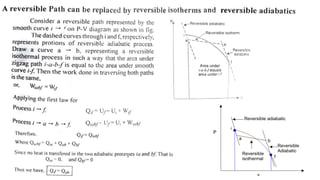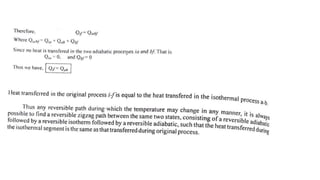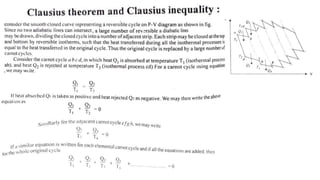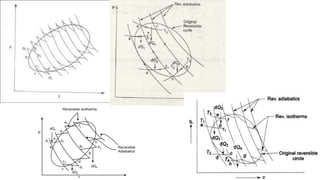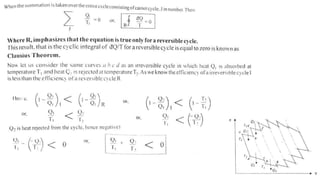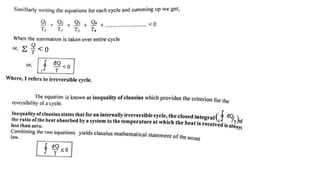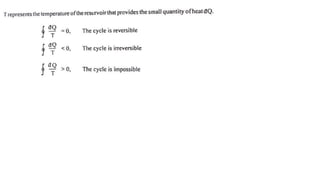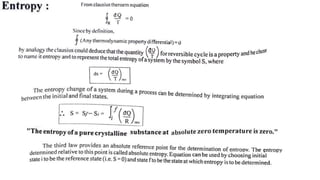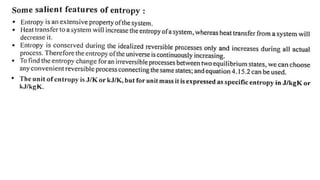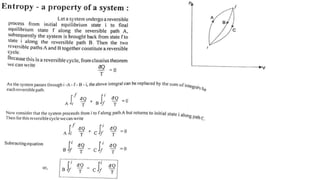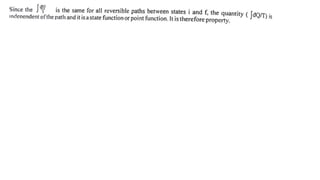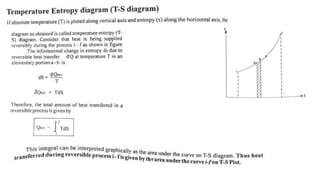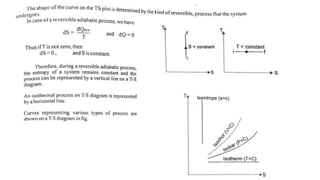1 von 45

### Basic mechanical engineering(BMET-101/102) unit 2 (part-2) thermodynamics laws by varun pratap singh

• 1. Basic Mechanical Engineering UNIT-2 Thermodynamics' Laws Part-Two (Second Law of Thermodynamics) By Mr. Varun Pratap Singh
• 6. Source Sink
• 7. In thermodynamics and engineering, a heat engine is a system that converts heat to mechanical energy, which can then be used to do mechanical work. It does this by bringing a working substance from a higher state temperature to a lower state temperature.
• 9. Reversible Heat Engine Every reversible heat engine operating between the same two temperature reservoirs have identical efficiency. This means no matter how a reversible heat engine is constructed or what the working fluid is, its efficiency is the same as all other heat engines working from the same two temperatures.
• 15. The Kelvin-Planck statement is the statement of the second law of thermodynamics for heat engines, while the Clausius statement is the statement of the refrigerators and heat pumps.
• 19. Equivalence of Kelvin-Planck and Clausius Statements The Clausius and Kelvin-Planck statements of the second law are entirely equivalent. This equivalence can be demonstrated by showing that the violation of either statement can result in violation of the other one.
• 21. The Clausius and Kelvin-Planck statements of the second law are entirely equivalent. This equivalence can be demonstrated by showing that the violation of either statement can result in violation of the other one.
• 28. CARNOT THEOREM AND COROLLARY